EnchantedLearning.com is a user-supported site.
As a bonus, site members have access to a banner-ad-free version of the site, with print-friendly pages.

 You might also like: C: Illustrated Math Dictionary - Enchanted Learning.com Today's featured page: School Words Book, A Printable Book

 +, - EnchantedLearning.comMath Dictionary x, ÷
 A B C D E F G H I J K L M N O P Q R S T U V W X Y ZT is for ...Figure Area
Squarea * a
Rectangle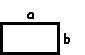a * b
Circle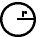πr 2

table

A table is an organized way of listing data in rows and columns.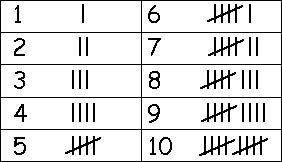tally marks

Tally marks are a quick way of keeping track of numbers in groups of five. One vertical line is made for each of the first four numbers; the fifth number is represented by a diagonal line across the previous four.

tangent

Tangent (abbreviated tan) is a trigonometric ratio, corresponding to the length of the oppsite side divided by the length of the adjacent side of the right triangle.tangram

A tangram is a traditional Chinese puzzle that can be made into many shapes.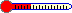temperatureTemperature is a measure of heat. Temperature is measured using a thermometer.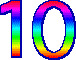tenTen is the number between nine and eleven. People have ten fingers and ten toes. Our number system is base 10. A ten-sided polygon is called a decagon. termsIn an expression or equation, terms are numbers, variables, or numbers with variables. For example, the expression 3x has one term, the expression 4x2 + 7 has two terms.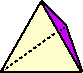tetrahedronA tetrahedron is a pyramid formed by four triangles.thermometerA thermometer is used for measuring temperature.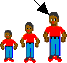thirdThe third person or item comes after the first and second. Third is abbreviated 3rd. 13*************thirteenThirteen is the number after twelve (12) and before fourteen (14). A thirteen-sided polygon is called a tridecagon. 30******************************thirtyThirty is the number after twenty nine (29) and before thirty one (31). A thirty-sided polygon is called an triacontagon.
 1,000thousandOne thousand is a whole number that is equal to 100 times 10.threeThree is the number between two and four. Tri means three. A tricycle has three wheels. A triangle has three sides. A Triceratops is a dinosaur that had three horns.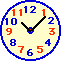timeTime is measured in seconds, minutes, hours, days, weeks, months, and years.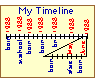timelineA timeline is a graphic display that shows a series of events that happen in a time period.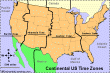time zoneThe Earth is divided into 24 time zones so that everyone in the world can be on roughly similar schedules (like having noon occur when the sun is highest in the sky).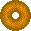torusA torus is a doughnut-shaped solid object. transcendental numberA transcendental number is a number that is not the root of a polynomial equation with integer coefficients. Examples of transcendental numbers include e and pi . transversalA transversal is a line that intersects at least two other lines.trapezoidA trapezoid is a four-sided figure (a quadrilateral) with exactly two parallel sides.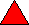triangleA triangle is a geometric figure (a polygon) that has three sides. The sum of the interior angles of a triangle is 180 degrees. The perimeter of a triangle is the sum of the lengths of the three sides. The area of a triangle is (1/2)base x height. A triangle with three equal sides (and three equal angles) is called an equilateral triangle. A triangle with two equal sides (and two equal angles) is called an isosceles triangle. A triangle with no equal sides (and no equal angles) is called an scalene triangle. A triangle with one right angle is called a right triangle. The Pythagorean theorem states that for a right triangle, the sum of the squares of the two shorter sides is equal to the square of the hypotenuse: a2 + b2 = h2. A triangle with one obtuse angle is called a obtuse triangle. A triangle with all acute angles is called a acute triangle. 2x + 3y - 5ztrinomialA trinomial is a polynomial expression that has three terms, like 3x2 + 4yz - 5.
 trisectTo trisect is to divide into three equal parts. For example, if a 90-degree angle is trisected, the result is three 30-degree angles. 12************twelveTwelve (12) is the number between eleven (11) and thirteen (13). Dozen is another word for twelve. A dodecagon is a twelve-sided figure. A dodecahedron is a twelve-sided geometric solid whose faces are pentagons.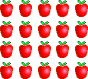twentyTwenty is the number between nineteen and twenty-one. There are twenty (20) apples above. A twenty-sided polygon is called an icosagon.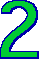twoTwo is the number between one and three. We have two eyes, two ears, two arms, and two legs.

 +, - EnchantedLearning.comMath Dictionary x, ÷
 A B C D E F G H I J K L M N O P Q R S T U V W X Y Z
 Number Line Fractions Decimals Graphs Measurement Rounding

Click on an underlined word for more information on that subject. If the math term you are looking for is not in the dictionary, please e-mail us.

Enchanted Learning®
Over 35,000 Web Pages
Sample Pages for Prospective Subscribers, or click below

 Overview of Site What's New Enchanted Learning Home Monthly Activity Calendar Books to Print Site Index K-3 Crafts K-3 Themes Little ExplorersPicture dictionary PreK/K Activities Rebus Rhymes Stories Writing Cloze Activities Essay Topics Newspaper Writing Activities Parts of Speech Fiction The Test of Time iPhone app TapQuiz Maps - free iPhone Geography Game Biology Animal Printouts Biology Label Printouts Biomes Birds Butterflies Dinosaurs Food Chain Human Anatomy Mammals Plants Rainforests Sharks Whales Physical Sciences: K-12 Astronomy The Earth Geology Hurricanes Landforms Oceans Tsunami Volcano Languages Dutch French German Italian Japanese (Romaji) Portuguese Spanish Swedish Geography/History Explorers Flags Geography Inventors US History Other Topics Art and Artists Calendars College Finder Crafts Graphic Organizers Label Me! Printouts Math Music Word Wheels

## Enchanted Learning Search

 Search the Enchanted Learning website for: